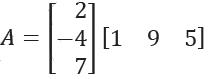Related Articles

# GATE | GATE-CS-2014-(Set-2) | Question 14

• Last Updated : 20 Jun, 2017

If the matrix A is such thatAttention reader! Don’t stop learning now.  Practice GATE exam well before the actual exam with the subject-wise and overall quizzes available in GATE Test Series Course.

Learn all GATE CS concepts with Free Live Classes on our youtube channel.

then the determinant of A is equal to
(A) 0
(B) 1
(C) 2
(D) 3

Explanation: This is a numerical answer question of gate paper, in which no options are provided, and the answer is to given by filling a numeral into a text box provided.

In the question, matrix A is given as the product of 2 matrices which are of order 3 x 1 and 1 x 3 respectively. So after multiplication of these matrices, matrix A would be a square matrix of order 3 x 3.

So, matrix A is :

2 18 10
-4 -36 -20
7 63 35

Now, we can observe by looking at the matrix that row 2 can be made completely zero by using row 1, this is to be done by using the row operation of matrix which here is :

R2 <- R2 + 2R1

After applying above row operation in the matrix, the resultant matrix would be:

2 18 10
0 0 0
7 63 35

i.e. Row 2 has become zero now. And if a square matrix has a row or column with all its elements as 0, then its determinant is 0. ( A property of a square matrix )